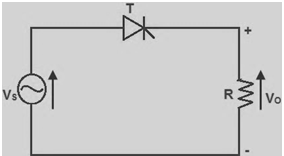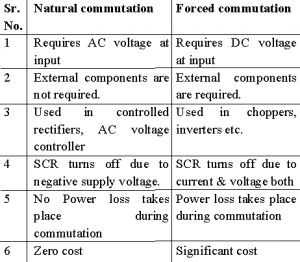Home > thyristor > thyristor commutation

# Thyristor Commutation

Concept of commutation

As we know that a thyristor can be turned on by triggering gate terminal with low voltage short duration pulse. But after turning on, it will conduct continuous until the thyristor is reverse biased or the load current falls to zero. This continuous conduction of thyristors causes problems in some applications. The process used for turning off a thyristor is called as commutation. By the commutation process, the thyristor operating mode is changed from forward conducting mode to forward blocking mode. So, the thyristor commutation methods or thyristor commutation techniques are used to turn off.
The commutation techniques of thyristors are classified into two types:
1. Natural Commutation
2. Forced Commutation

• Natural Commutation (Line Commutation)
Generally, if we consider AC supply, the current will flow through the zero crossing line while going from positive peak to negative peak. Thus, a reverse voltage will appear across the device simultaneously, which will turn off the thyristor immediately. This process is called as natural commutation as thyristor is turned off naturally without using any external components or circuit or supply for commutation purpose. Figure below shows the Natural Commutation. Natural commutation can be observed in AC voltage controllers, phase controlled rectifiers and cycloconverters.• Forced Commutation
The thyristor can be turned off by reverse biasing the SCR or by using active or passive components. Thyristor current can be reduced to a value below the value of holding current. Since, the thyristor is turned off forcibly it is termed as a forced commutation process. The basic electronics and electrical components such as inductance and capacitance are used as commutating elements for commutation purpose. Forced commutation can be observed while using DC supply; hence it is also called as DC commutation. The external circuit used for forced commutation process is called as commutation circuit and the elements used in this circuit are called as commutating elements.
The Forced commutation is classified as-
a) Voltage commutation
b) Current commutation
Forced commutation requires external components to store energy & used to apply reverse voltage across SCR or reduce anode current below holding current.
• Current commutation-SCR is turned off by reducing anode current below holding current.
• Voltage commutation-SCR is turned off by applying large reverse voltage across it.

Classification of Forced Commutation Methods.
The forced commutation can be classified into different methods as follows:
1. Class A: Self commutated by a resonating load
2. Class B: Self commutated by an LC circuit
3. Class C: Cor L-C switched by another load carrying SCR
4. Class D: C or L-C switched by an auxiliary SCR
5. Class E: An external pulse source for commutation
6. Class F: AC line commutation

Natural Vs Forced communication